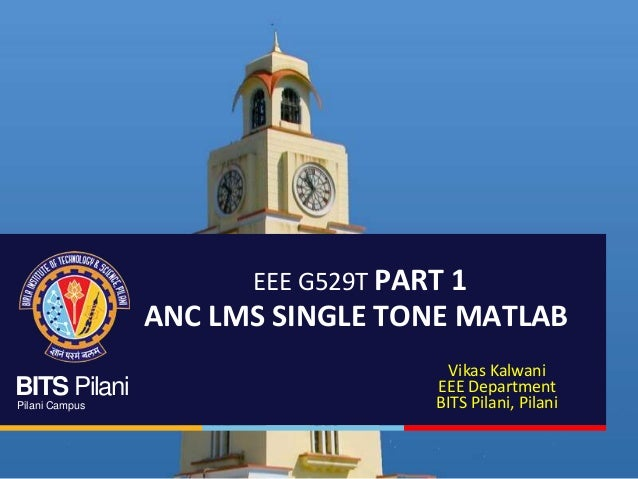Successfully reported this slideshow.Upcoming SlideShare
×

# ACTIVE NOISE CONTROL FOR A SINGLE TONE

580 views

Published on

ACTIVE NOISE CONTROL by means of an adaptive filter that filters out white gaussian noise from a single tone sine wave.
LMS Algorithm is used to change the filter weights of the Adaptive filter. The project has been implemented in Matlab.

Published in: Technology
• Full Name
Comment goes here.

Are you sure you want to Yes No• Be the first to like this

### ACTIVE NOISE CONTROL FOR A SINGLE TONE

1. 1. BITS Pilani Pilani Campus EEE G529T PART 1 ANC LMS SINGLE TONE MATLAB Vikas Kalwani EEE Department BITS Pilani, Pilani
2. 2. BITS Pilani, Pilani Campus ACTIVE NOISE CONTROL Why do we need Noise Control?  Noise control is a very important aspect of modern engineering systems  In aircrafts, control on the engine noise is a must for the Air Traffic Industry to Flourish  In the offices of MNC’s that work in the urban Residency amidst traffic and industrial noises, Noise control is essential  Also, many biological problems due to Noise when uncontrolled Types of Noise Control ACTIVE PASSIVE • Uses Active devices • Efficient at low frequencies • Selective Filtering • Less Bulky • Uses Passive elements like Thermocol etc. to block the path of the noise thereby attenuating it
3. 3. BITS Pilani, Pilani Campus BASICS Primary Input -> Signal s + Noise n The Noise is not correlated to the signal Reference Input -> Noise that is correlated to the noise present in the primary signal. Reference signal can be recorded near the noise source or when the signal is not present.  The Reference Input is filtered by the Adaptive filter to ŋ which is a close approximate to the noise in the primary input . The filtered output ŋ is subtracted from the primary input to produce the System Output. The adaptive algorithm updates the filter coefficients of the filter based on this System Output. The Output is processed by the LMS Algorithm which in turn changes the weights of the filter. NOTE Useful signal s is uncorrelated with n and ŋ. E[sn]=0 and E[sŋ]=0
4. 4. BITS Pilani, Pilani Campus  Subtracting noise from a signal involves the risk of distorting the signal and if done improperly, may lead to increase in the noise level. So, the noise estimate ŋ should be an exact copy of n  characteristics of the transmission paths are unknown and unpredictable, filtering and subtraction are controlled by an adaptive process. Hence, an adaptive filter capable of adjusting its impulse response to minimize an error signal (which depends on the filter output) is used.  Error Signal/System Output is minimized using LMS, RLS, NLMS and other algorithms
5. 5. BITS Pilani, Pilani Campus ADAPTIVE NOISE CANCELLER In noise canceling systems the objective is to produce a system output ŝ = s + n - ŋ that is a best fit in least square sense to signal s. This is done by feeding the system output back to the adaptive filter and adjusting the filter through adaptive algorithm to minimize total system output power i.e. system output serves as error signal for adaptive process.
6. 6. BITS Pilani, Pilani Campus ŝ = s + n – ŋ ŝ2 = s2 + (n – ŋ)2 + 2s(n – ŋ) • Taking expectations on both sides, E[ŝ2]= E[s2]+ E[(n – ŋ)2] + E[2s(n – ŋ)] • Realizing that s is uncorrelated to n and ŋ, E[2s(n – ŋ)]=0 E[ŝ2]= E[s2]+ E[(n – ŋ)2] • The signal power E[s2] remains unaffected as the filter is adjusted to minimize E[ŝ2] min(E[ŝ2])= E[s2]+ min(E[(n – ŋ)2]) • As signal power is fixed and noise power of the output is getting reduced, we get higher signal to noise ratios To be minimized E[x2(t)] = RMS POWER NOISE CANCELLATION Signal power
7. 7. BITS Pilani, Pilani Campus LMS ALGORITHM
8. 8. BITS Pilani, Pilani Campus SIMULATION AND RESULTS LMS Algorithm was implemented successfully. The following graphs describe it. TIME DOMAIN REPRESENTATION R=10 (Repetitions) I=50000 (Iterations) L=5 (Length) N=4 (Order) u=0.002 (Step Size Parameter) PARAMETERS
9. 9. BITS Pilani, Pilani Campus SIMULATION AND RESULTS Mean Square Error (dB) The MSE reduces by a margin of 25 dB. We can have a faster reduction in MSE by increasing the value of step Size parameter u.
10. 10. BITS Pilani, Pilani Campus VARIATION IN PARAMETERS VARIATION IN NUMBER OF ITERATIONS I=10000 I=50000 Noise Source Signal v = 0.8*randn(I,1); More reduction in MSE when the number of Iterations are large [MSE(dB)]10000 = -20.64 dB [MSE(dB)]50000 = -27.27 dB
11. 11. BITS Pilani, Pilani Campus VARIATION IN PARAMETERS VARIATION IN NUMBER OF ITERATIONS I=10000 I=50000 Noise Source Signal v = 0.6*randn(I,1); More reduction in MSE when the number of Iterations are large [MSE(dB)]10000 = -13.71 dB [MSE(dB)]50000 = -27.27 dB u=0.0002
12. 12. BITS Pilani, Pilani Campus VARIATION IN PARAMETERS VARIATION IN LENGTH OF ADAPTIVE FILTER L=5 L=10 Noise Source Signal v = 0.8*randn(I,1); More reduction in MSE when length of the AF is larger [MSE(dB)]5 = -29.72 dB [MSE(dB)]10 = -40.28 dB u=0.0002 I=50000
13. 13. BITS Pilani, Pilani Campus VARIATION IN PARAMETERS VARIATION IN STEP SIZE PARAMETER u=0.0002 Noise Source Signal v = 0.8*randn(I,1); Quicker reduction in MSE when u is larger [MSE(dB)]5 = -29.72 dB [MSE(dB)]10 = -29.23 dB I=50000 L=5 u=0.001
14. 14. BITS Pilani, Pilani Campus ANC has been done for Single Tone Signal here. Real Test begins, when the signal is a speech signal. For that we will use the LMS Block in the Data Acquisition Toolbox of Matlab.